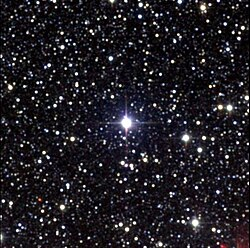Tags

Relativity is mind-bending. Rightly so, Einstein and his equations represent some of the most amazing advances in modern physics. But fundamentally, many of these counter-intuitive space-time properties can be derived with simple thought experiments and some algebra. Of course, discovering that time moves differently for different observers at different velocities takes a flash of insight that few of us have, but at least we can proceed from there.

The fact that the speed of light is the same in all reference frames has the consequence that moving clocks run slow. This stunning fact applies to time itself; astronauts orbiting the earth at speed biologically age more slowly than the rest of us. So, if two events occur at the same place, such as the ticks of a clock, a moving observer will measure the time between the events to be longer.

We can start deriving how time differs for stationary and moving observers by considering a light clock. This imaginary clock would work by ticking every time a light pulse (a photon moving at the speed of light) reflected back to the lower mirror as shown below:In the diagram, “h” represents the vertical distance between the mirrors. The stationary observer here observes a particular time, but what about a moving observer? The diagram below shows the change:Here we have moving mirrors with the photon moving at the same speed and hitting the same place on the mirrors (so that it would be identical to the first diagram if the mirrors were not moving). All the parameters here are the same, expect for the movement, and relativity states that then the time will change. Let’s derive that change for ourselves, no Einstein required. I have outlined each step in the necessary tedium below:There you have it! With some simple algebra and a clever thought experiment, we have derived ourselves how time changes as you move closer and closer to the speed of light.

Let’s give it a quick test run. Suppose that we wish to get to the nearest star beyond our sun, Proxima Centauri.Proxima Centauri (center inset) as seen by 2MASS

Proxima Centauri is 4.24 light years away from Earth. The speed of light is around 186,282 miles per second (299,792,458 meters per second). This means that the star is nearly 25,000,000,000,000 miles away (38,000,000,000,000 kilometers).

To get there, we could use one of the fastest spacecraft ever built:

The figure always cited here is the heliocentric speed of Voyager 1, some 17.05 kilometers per second, which is faster than any of our outward bound spacecraft…

At this speed, it would take us over 70,000 years to get there! But what if we could move fast enough to make our equation that we derived above matter? What if we could move at 30% the speed of light? How long would that take us?

At 30% the speed of light, our trip would shorten to only 14 years. But time dilation then becomes a factor:This means that in our ship we would be .65 years or around 8 months younger than people back on Earth when we reached the star! And this effect get exponentially larger as we get closer to the speed of light, as seen in the graph below:For example, at 99% the speed of light, for our 14 year journey people on Earth would age 85 years more than us!By the time we returned from our journey, because of time dilation, everyone we knew would have long since died. This is the peril of long-distance space travel.

And all this from a mirror-clock and some algebra.